ISEE Middle Level Math : How to find the square root

Example Questions

Example Question #271 : Isee Middle Level (Grades 7 8) Mathematics Achievement

Solve: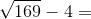Explanation:

First find the square root: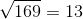Then subtract: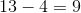Answer:Example Question #272 : Isee Middle Level (Grades 7 8) Mathematics Achievement

Solve: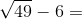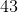Explanation:

First find the square root: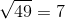Then subtract: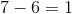Answer:Example Question #273 : Isee Middle Level (Grades 7 8) Mathematics Achievement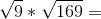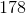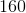Explanation:

First find the square roots: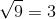Then multiply: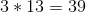Answer:Example Question #274 : Isee Middle Level (Grades 7 8) Mathematics Achievement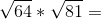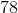Explanation:

First find the square roots: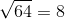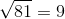Then multiply: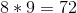Answer:Example Question #275 : Isee Middle Level (Grades 7 8) Mathematics Achievement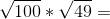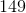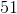Explanation:

First find the square roots: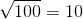Then multiply: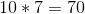Answer:Example Question #276 : Isee Middle Level (Grades 7 8) Mathematics Achievement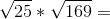Explanation:

First find the square roots: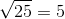Then find the product: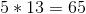Answer:Example Question #277 : Isee Middle Level (Grades 7 8) Mathematics Achievement

Solve: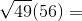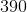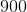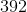Explanation:

First, find the square root:Then find the product: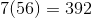Answer:Example Question #278 : Isee Middle Level (Grades 7 8) Mathematics Achievement

Solve: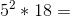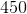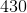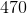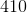Explanation:

First square 5: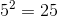Then multiply: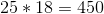Answer:Example Question #279 : Isee Middle Level (Grades 7 8) Mathematics Achievement

Evaluate: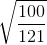is undefined.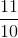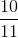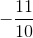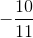Explanation:

To find the square root of a fraction, extract the square root of both the numerator and the denominator. Since, and since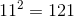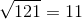. and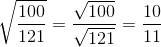Example Question #280 : Isee Middle Level (Grades 7 8) Mathematics Achievement

Evaluate: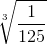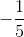is undefined.To find the cube root of a fraction, extract the cube root of both the numerator and the denominator. Since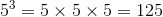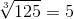, and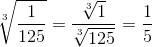.